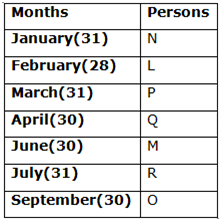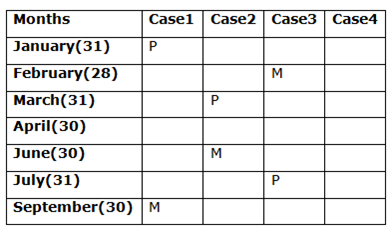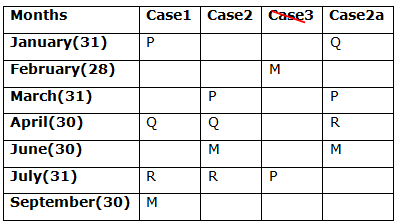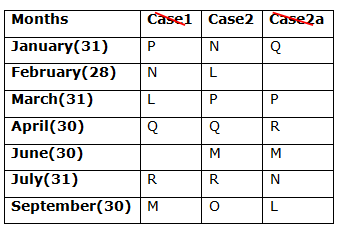# IBPS Clerk Prelims Reasoning (Day-17)

Dear Aspirants, Our IBPS Guide team is providing new series of Reasoning Questions for IBPS Clerk Prelims 2020 so the aspirants can practice it on a daily basis. These questions are framed by our skilled experts after understanding your needs thoroughly. Aspirants can practice these new series questions daily to familiarize with the exact exam pattern and make your preparation effective.

Start Quiz

Month based puzzle

Directions (1-5): Study the following information carefully and answer the below questions.

Seven people L, M, N, O, P, Q, and R are joining a company in different month viz., January, February, March, April, June, July, and September but not necessarily in the same order.

P joins in the month which has 31 days. R joins three months after Q. O joins the adjacent month of neither Q nor P. There are as many persons join before P as after M. L joins just after N. R joins either immediately before or immediately after M.

1) How many persons were joined between N and the one who joined in June?

a) Two

b) Three

c) Four

d) Five

e) None

2) Four of the following five are alike in a certain way and hence form a group. Who among the following one does not belong to the group?

a) NL

b) PQ

c) MR

d) QM

e) NO

3) The number of persons joined before L is one less than the number of persons joined after ____?

a) P

b) The one who joined in July

c) L

d) The one who joined in June

e) Q

4) Who among the following person was joined after L but before Q?

a) M

b) N

c) The one who joined in March

d) The one who joined in April

e) None

5) In a certain way, N is related to Q in the same way P is related to R then who among the following person is related to O?

a) R

b) M

c) L

d) Q

e) None

Inequality

Directions (6-10): In each of the following questions, the relationship between different elements is shown in the statements followed by five conclusions. Find a conclusion which logically follows.

6) Statements:

L ≥ M > N < O ≤ X = V

Conclusions:

a) L ≥ N

b) O ≤ V

c) M < O

d) X ≤ M

e) None of the above

7) Statements:

Z ≥ N <  P; C = V ≥ R < P

Conclusions:

a) N < R

b) P ≤ C

c) Z ≥ V

d) N > C

e) None of these

8) Statements:

R > T; N > L; Z ≤ T; L > Q = R

Conclusions:

a) N ≥ Z

b) T > L

c) Q = T

d) N > R

e) None of these

9) Statements:

B ≤ D = N > K ≤ A < C

Conclusions:

a) D < C

b) D ≥ A

c) B ≤ A

d) K < C

e) C > N

10) Statements:

E ≤ F > P > S ≥ T = O ≤ L

Conclusions:

a) F > T

b) P ≥ O

c) E ≤ T

d) L > F

e) S > O

Directions (1-5) :P joins in the month which has 31 days.

There are as many persons join before P as after M.

From the above condition, there are three possibilities.R joins three months after Q.

R joins either immediately before or immediately after M.

Case-3 gets eliminated because we have no place for Q.L joins just after N.

O joins the adjacent month of neither Q nor P.

Case-1 and Case-2a gets eliminated Since O joins an adjacent month of neither Q nor P.So Final arrangement becomes,Directions (6-10) :

O ≤ X = V

N > L >  Q = R > T ≥ Z

K ≤ A < C